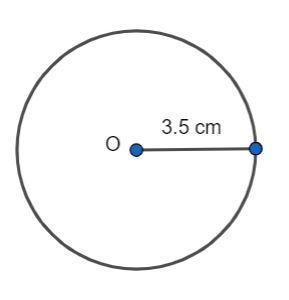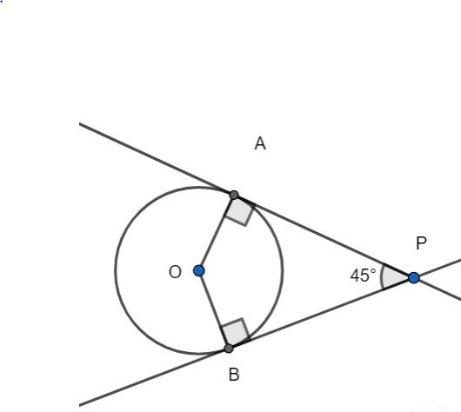QUESTION

# Draw a circle with center O and radius 3.5 cm. Draw two tangents PA and PB from an external point P such that $\angle APB = 45^\circ$. What is the value of $\angle AOB + \angle APB$?

Hint: A circle is uniquely drawn when its center and radius are given. The tangents and the radius are perpendicular to each other at the point of contact of the tangent. The sum of the angles in a quadrilateral is 360°. Use these facts to find the sum $\angle AOB + \angle APB$.

The center of the circle is the fixed point from which all points on the circle are equidistant. The distance between the center of the circle and any point on it is called its radius.
We draw the circle with center O and radius 3.5 cm as shown in the figure.From an external point P, we draw two tangents to the circle, PA and PB, such that the angle APB is 45°.The tangents drawn from the external point are equidistant from the circle and the radius is perpendicular to the tangent at the point of contact of the tangent on the circle. Hence, we have:
$\angle PAO = 90^\circ .............(1)$
$\angle PBO = 90^\circ .............(2)$
We know that the sum of the interior angles of a quadrilateral is 360°. Then, for the quadrilateral PAOB, we have:
$\angle PAO + \angle PBO + \angle AOB + \angle APB = 360^\circ$
Using equations (1) and (2), we have:
$90^\circ + 90^\circ + \angle AOB + 45^\circ = 360^\circ$
Simplifying, we have:
$\angle AOB + 225^\circ = 360^\circ$
Solving for the angle AOB, we have:
$\angle AOB = 360^\circ - 225^\circ$
$\angle AOB = 135^\circ ............(3)$
The sum of the angles AOB and APB is given as follows:
$\angle AOB + \angle APB = 135^\circ + 45^\circ$
$\angle AOB + \angle APB = 180^\circ$
Hence, the required sum of the angles is 180°.

Note: You need not necessarily find the angle AOB to find the sum of the angles, you can directly find the sum from the property of the angles of the quadrilateral.